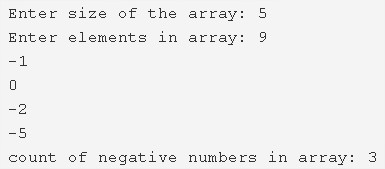# C Program To Count Number Of Negative Elements In Array

C program to count the total number of negative elements in an array – In this article, we will explain the multiple means to count the total number of negative elements in an array.

Suitable examples and sample programs have also been added so that you can understand the whole thing very clearly. The compiler has also been added with which you can execute it yourself.

The means used in this distinct piece are as follows:

• Using Standard Method
• Using Function
• Using Recursion

As we all know, in an array, a bunch of elements are placed in any particular order, be it positive or negative elements.

Arrays are a means of showcasing the stored information in a specific pattern.As you can see in the photo uploaded above, firstly, you have to enter the size of the array.

In this case, the size of the array is mentioned as 5.

Later on, the elements are also added accordingly.

The elements for this particular array are 9, -1, 0, -2, -5.

As we can see, there are three negative elements.

Those are -1, -2, -5.

Hence, the number or the count of the negative numbers in the array = 3.

Thus, the methods used to do the same in C programming are as follows:

## Using Standard Method

1. c=0, Read the size of the array and store that value into the variable n.

2) Read the entered array elements using scanf() predefined function and store the elements into the array using for loop for(i=0;i<n;i++).

3) Compare each element of the array with zero as a[i]<0,

a) If any element is less than zero then increase the count value c by 1.Repeat until all iterations of for loop for(i=0; i<n;i++).

4) Print the count value c which is the count of negative numbers in the array.

Output:

### Using Function

1. The function countnegativenumbers() is the user-defined function which return the number of negative numbers in the array to the main() function.

2) The main() function calls the countnegativenumbers() function by passing array,array size as arguments.

3) The function countnegativenumbers() compares each element of the array with zero as a[i]<0. If any element is less than zero then it increases the count c value by 1. After all the iterations of “for” loop, it returns the c value to the main() function.

4) The function main() prints the count value c which represent the total negative numbers in an array.

Output:

#### Using Recursion

1. The main() calls the countnegativenumbers() function by passing array, size of the array,i=0 as arguments.

2) The function countnegativenumbers() checks the condition i<n. If this condition is true then

a) Itcompare the each array element with zero as a[i]<0. If it is true then c value increased by 1.

b) This function calls itself by increasing i value by 1 until the condition i<n becomes false. Here the function countnegativenumbers() is the recursive function.

This function returns c value to main(), main() function prints the count value.

Output:
x

## C Program Inverted Pyramid Star Pattern | 4 Ways – C Programs

C Program to print an inverted pyramid star pattern – In this article, we will ...